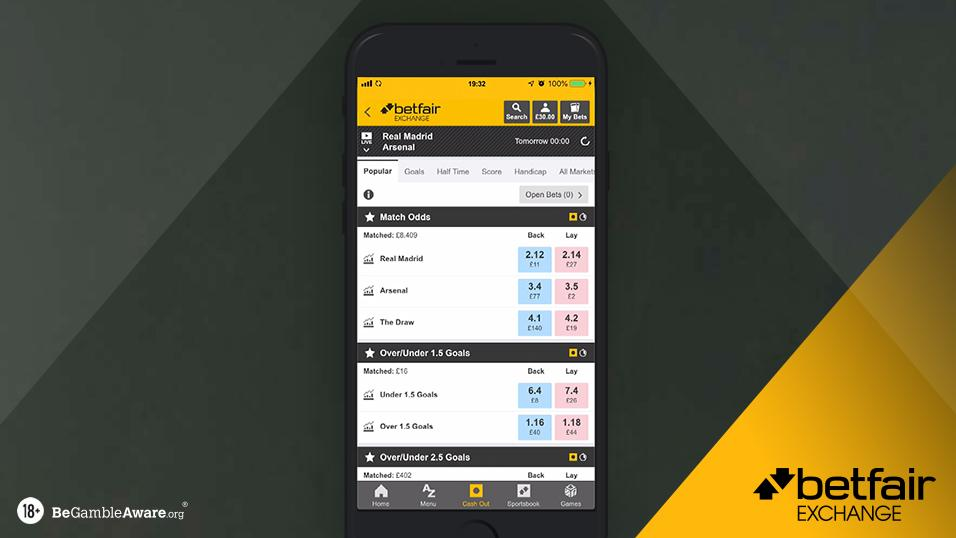# Understanding Decimal OddsWhen placing a bet, your odds will be displayed in one of two ways, fractions or decimals, and many betting websites, including the Betfair Exchange, now use decimal odds as standard, so it’s important to understand how it works.

Decimal odds are popular because they are easy to understand, displaying the total return from a 1 unit stake. They allow you to easily calculate how much money you will be returned if your bet wins. To work this out, simply multiply your stake by the decimal number displayed and that is how much you will receive, plus your stake. As the unit stake is always included, decimal odds always have a value greater than 1.

The total (potential) return on a stake can be calculated as:

Total Return = Stake x Decimal Odd Number

The decimal odds will be displayed up to 2 decimal places, but the return will be paid out by calculating at the actual odds, to the nearest £/€ 0.01. For example, if your bet is placed at odds of 4.3333333 (this is 10/3 in fractional), a £/€ 10 bet would return £/€ 43.33 if it wins, with your odds shows as 4.33.

Examples:

9.00 - for every £/€1 you bet, you will win £/€8. You will also receive your £/€1 stake back so your total return is £/€9.

2.00 - for every £/€1 you bet, you will win £/€1. You will also receive your £/€1 stake back so your total return is £/€2.

1.25 - for every £/€1 you bet, you will win £/€0.25. You will also receive your £/€1 stake back so your total return is £/€1.25.

Punters in the UK and Ireland may not be as comfortable with decimal odds, though decimals are a common sight at racecourses across the UK & Ireland thanks to the Tote, because fractional odds are more commonly used, however they can sometimes be confusing as the calculation can produce very large numbers. For example, where the decimal odds are 1.46, the fractional equivalent would be 23/50. In this case, it is much easier to calculate the return from a £1 stake by using the decimal odds format.

Also, fractional odds can be confusing when the probability is very high. For example, when decimal odds fall below 2, the fractional equivalent suddenly takes a different appearance as the second number will be larger than the first number. Therefore, it is possible to have fractional odds of 2/1 and 1/2 and this could be confusing the novice punter.

If you want to partake in sports betting then understanding how to read odds is a vital part of the process. If you do not understand how to read the odds, you will never know if you are getting good value or what your potential returns might be.

Decimal odds are the easier of the two to understand and many punters have switched to using decimal odds out of choice after years of using the fractional format as they can be read at a glance and do not require any tricky calculations.

Converting fractional odds to decimal odds can be tricky, so below is a handy guide.